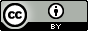# Schultz, T.W.; Applehans, F.M. Correlations for the acute toxicity of multiple nitrogen substituted aromatic molecules. Ecotoxicol. Environ. Saf. 1985, 10, 1, 75–85.

## QsarDB content

### Property pIGC50: 60-h Tetrahymena toxicity as log(1/IGC50) [log(L/mmol)]

##### 1: All compounds

Regression model (regression)

Open in:QDB Explorer QDB Predictor

Name Type n

R2

σ

Training training 29 0.324 0.410
##### 2: Aza-arenes

Regression model (regression)

Open in:QDB Explorer QDB Predictor

Name Type n

R2

σ

Training training 9 0.348 0.401
##### 3: Indoles and indole analogs

Regression model (regression)

Open in:QDB Explorer QDB Predictor

Name Type n

R2

σ

Training training 7 0.743 0.227
##### 4: Arenes

Regression model (regression)

Open in:QDB Explorer QDB Predictor

Name Type n

R2

σ

Training training 13 0.122 0.400
##### 5: Compounds with a single polar group

Regression model (regression)

Open in:QDB Explorer QDB Predictor

Name Type n

R2

σ

Training training 14 0.515 0.329
##### 6: Compounds with multiple polar groups

Regression model (regression)

Open in:QDB Explorer QDB Predictor

Name Type n

R2

σ

Training training 15 0.194 0.376
##### 7: Compounds with ortho- or para-oriented groups

Regression model (regression)

Open in:QDB Explorer QDB Predictor

Name Type n

R2

σ

Training training 11 0.889 0.185
##### 8: Compounds with meta-oriented groups

Regression model (regression)

Open in:QDB Explorer QDB Predictor

Name Type n

R2

σ

Training training 18 0.611 0.275
##### 9: First meta-substituted subset

Regression model (regression)

Open in:QDB Explorer QDB Predictor

Name Type n

R2

σ

Training training 11 0.845 0.205
##### 10: Second meta-substituted subset

Regression model (regression)

Open in:QDB Explorer QDB Predictor

Name Type n

R2

σ

Training training 7 0.965 0.053

## Citing

When using this QDB archive, please cite (see details) it together with the original article:

 Title: Schultz, T.W.; Applehans, F.M. Correlations for the acute toxicity of multiple nitrogen substituted aromatic molecules. Ecotoxicol. Environ. Saf. 1985, 10, 1, 75–85. Abstract: Twenty-nine compounds, including amino and nitro derivatives of aromatic hydrocarbons as well as azaarenes and indole analogs, were assayed. The biological activity was monitored as population growth to axenic cultures of the ciliate Tetrahymena pyriformis. Structure-activity relationships were examined by linear regression analysis of log biological activity (Y) and log 1-octanol/water partition coefficient (X). Partition coefficients were determined by linear regression analysis of the retention time index of HPLC data. Attempts to generate linear predictive correlations for all the test compounds (n = 29; r2 = 0.325; P greater than 0.0013) and groups, subdivided based on the following chemical classes: aromatic hydrocarbons (n = 13; r2 = 0.122; P greater than 0.2417), azaarenes (n = 9; r2 = 0.348; P greater than 0.0946) and indole analogs (n = 7; r2 = 0.743; P greater than 0.0126), met with little success. Similarly, subdivisions based on the presence of a single (n = 14; r2 = 0.514; P greater than 0.0039) or multiple H-polar groups, i.e., NO2, NH2 (n = 15; r2 = 0.195; P greater than 0.0996), met with little success also. However, if one segregates the compounds which have para oriented H-polar substituents one gets a strong linear predictive correlation (n = 11; r2 = 0.889; P greater than 0.0001). A subdivision of the remaining data set leads to two models (n = 11; r2 = 0.845; P greater than 0.0001) and (n = 7; r2 = 0.965; P greater than 0.0001). An examination of the toxicity partitioning product values suggests the three-line predictive correlation model of these data is valid. URI: http://hdl.handle.net/10967/22 http://dx.doi.org/10.15152/QDB.22 Date: 2012-05-23
﻿

## Files in this item

Name Description Format Size View
0147651385900090.qdb.zip n/a application/zip 11.62Kb View/OpenFiles associated with this item are distributed